# Color Matching

Learn about how light is combined to make color in this color matching game. A random color slows creeps towards you. You need to match the incoming color by pressing the red, green, and blue buttons and rotating the knob.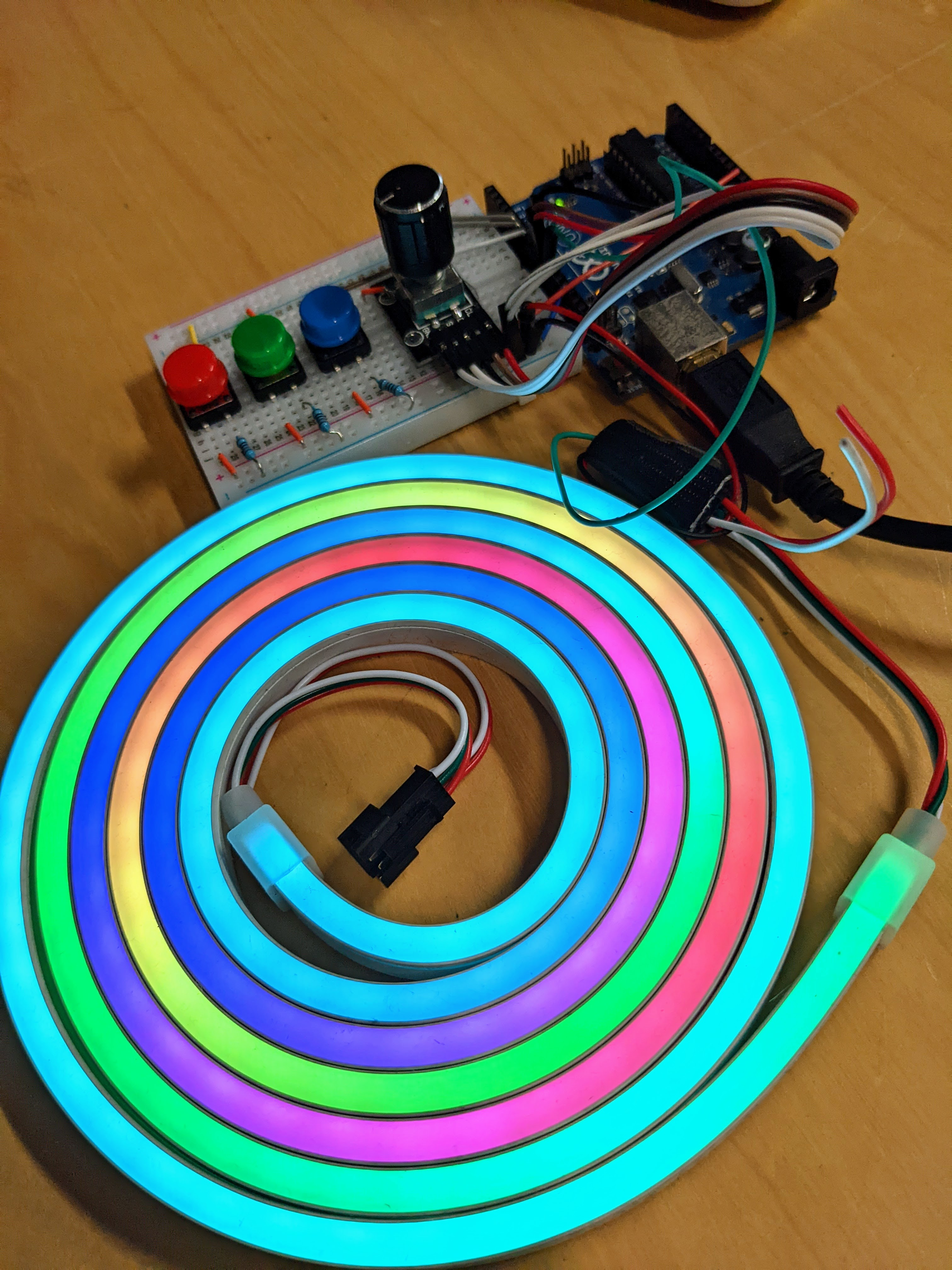more pictures: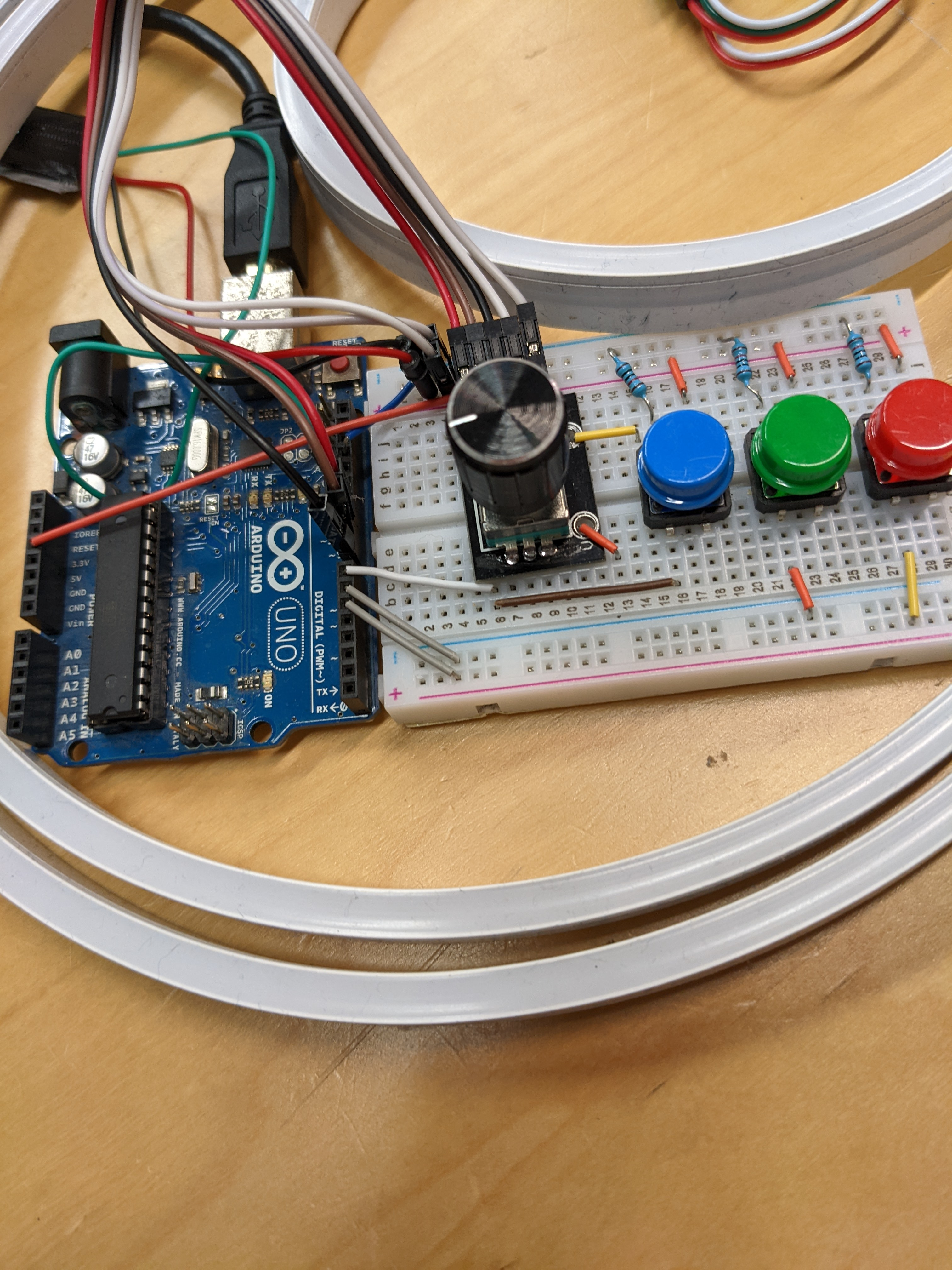The lights are LED strips controlled by an Arduino Uno using the NeoPixels library. They have a layer of silicon above 192 densely spaced addressable LEDs. The silicon diffuses the light to make the strip look like a solid bar of color.

A random line of color creeps towards the end of the light strip. The player tried to generate a color that matches that color. Pressing the red, green, and blue buttons sets what color you want. Twisting the rotary encoder knob will either add or subtract that color from your guess.

Deciding if your color guess was a close enough match was difficult to code. First, your guess is converted from RGB into HSV (hue saturation value). Next, your guess hue and saturation are compared to the random target. If they are close enough the game starts over.

If your guess is too different from the random target color you "lose". After losing the game generates a rainbow pattern until you press a key to try again.

The code expects the rotary encoder RotaryEncoder.h, and neopixel Adafruit_NeoPixel.h libraries.

materials

• Arduino Uno microcontroller
• any neopixel light strip
• 3 buttons
• Rotary Encoders
• jumper wires
• code

### Github - colormatch.ino

``````#include <Adafruit_NeoPixel.h>
#include <Arduino.h>
#include <RotaryEncoder.h>
#define PIN_IN1 10
#define PIN_IN2 9
RotaryEncoder encoder(PIN_IN1, PIN_IN2, RotaryEncoder::LatchMode::TWO03);

#define PIN 11
#define NUMPIXELS 192
Adafruit_NeoPixel strip(NUMPIXELS, PIN, NEO_GRB + NEO_KHZ800);
#define MAXBRIGHTNESS 100

#define PIN_R_BUTTON 5
#define PIN_G_BUTTON 6
#define PIN_B_BUTTON 7
#define PIXEL_EDGE 9 //where the incoming color wave collides with your input colors
#define ROTARY_RATE 16 //how fast do the colors change when you twist the dial
#define PIXEL_GROW_RATE 0.07 //difficulty / how long do you have to match colors
#define COLOR_MATCH_THRESHOLD 75 //difficulty / how close your color needs to be to target

bool inputMode[] = {0, 0, 0};
float pixelTimer = 0;
int cycle = 0;
float R = 0, G = 0, B = 0;
int R_int = 0, G_int = 0, B_int = 0;
int target_sat = random(0, 255);
unsigned int target_hue = random(0, 65536);
uint32_t target_color = strip.gamma32(strip.ColorHSV(target_hue, target_sat, 255)); //(Hue 0 - 65536), (Sat0-255),(Value 0-255)
byte rgb;
double hsv;

void setup() {
// Serial.begin(9600);
strip.begin(); // INITIALIZE NeoPixel strip object (REQUIRED)
strip.setBrightness(MAXBRIGHTNESS);
pinMode(PIN_R_BUTTON, INPUT);
pinMode(PIN_G_BUTTON, INPUT);
pinMode(PIN_B_BUTTON, INPUT);
}

void loop() {
cycle++;
pixelTimer += PIXEL_GROW_RATE;
//check for color collision
if (pixelTimer > (NUMPIXELS - PIXEL_EDGE)) {
const uint32_t color = strip.getPixelColor(PIXEL_EDGE - 1);
rgb = min(MAXBRIGHTNESS, color >> 16 & 255); // red
rgb = min(MAXBRIGHTNESS, color >> 8 & 255); // green
rgb = min(MAXBRIGHTNESS, color & 255); // blue
RGBtoHSV(rgb, hsv);
const float taget_hue_360 = float(target_hue) / 65536 * 360; //convert to 360 hue
const int diff = mod(int(hsv - taget_hue_360 + 180), 360) - 180; //find difference between each color arc hue
// const int sat = abs(R_int - G_int) + abs(B_int - G_int) + abs(R_int - B_int);

//lose and reset
//check if too different from hue,too different in saturation, too low in value
if (abs(diff) + abs(hsv - target_sat) / 3 > COLOR_MATCH_THRESHOLD || hsv < 15) {
//death animation
for (int i = 0; i < PIXEL_EDGE; i++) {
// strip.setPixelColor(random(0,NUMPIXELS-1), 0,0,0);//random place on strip goes white
// target_hue += 1000;
// target_color = strip.gamma32(strip.ColorHSV(target_hue, target_sat, 0)); //(Hue 0 - 65536), (Sat0-255),(Value 0-255)
strip.setPixelColor(PIXEL_EDGE - i-1, 0,0,0);
strip.show();   // Send the updated pixel colors to the hardware.
delay(400);
}
cycle = 0;
idle();
delay(500);
} else { //survive and reset
for (int i = 0; i < NUMPIXELS; i += 3) {
strip.setPixelColor(i, 0, 0, 0);
strip.setPixelColor(i + 1, 0, 0, 0);
strip.setPixelColor(i + 2, 0, 0, 0);
strip.show();
}
}
strip.clear(); // Set all pixel colors to 'off'
strip.show();
//resetting
R = 0;
G = 0;
B = 0;
target_sat = random(0, 255);
target_hue = random(0, 65536);
target_color = strip.gamma32(strip.ColorHSV(target_hue, target_sat, 255)); //(Hue 0 - 65536), (Sat0-255),(Value 0-255)
pixelTimer = 0;
}

//check buttons and set color input mode
}

static int pos = 0;
encoder.tick();
int newPos = encoder.getPosition();
if (pos != newPos) {
pos = newPos;
const int direction = -(int)(encoder.getDirection());
if (inputMode) {
R += ROTARY_RATE * direction;
if (R > 255) R = 255;
if (R < 0) R = 0;
}
if (inputMode) {
G += ROTARY_RATE * direction;
if (G > 255) G = 255;
if (G < 0) G = 0;
}
if (inputMode) {
B += ROTARY_RATE * direction;
if (B > 255) B = 255;
if (B < 0) B = 0;
}
}

R_int = int(constrain(R, 0, 255));
G_int = int(constrain(G, 0, 255));
B_int = int(constrain(B, 0, 255));
for (int i = 0; i < 3; i++)  strip.setPixelColor(i, 255 * inputMode, 255 * inputMode, 255 * inputMode);
if (R_int + G_int + B_int > 0) {
rgb = R_int;
rgb = G_int;
rgb = B_int;
}
RGBtoHSV(rgb, hsv);
for (int i = 3; i < PIXEL_EDGE; i++)  strip.setPixelColor(i, strip.Color( R_int, G_int, B_int ));
strip.setPixelColor(NUMPIXELS - pixelTimer, target_color);
strip.show();   // Send the updated pixel colors to the hardware.
}

while (1) { //wait until player presses and releases a button
if (cycle > 255)   break;
for (int i = 0; i < NUMPIXELS; i++) {
const int a = i + cycle;
strip.setPixelColor(i, strip.gamma32(strip.ColorHSV(32768+65536*sin(a*0.02),min(255, 255 + 170*sin(cycle*0.01)), cycle))); //180+75*sin(a*0.1)
}
cycle++;
strip.show();   // Send the updated pixel colors to the hardware.
// delay(10);
}
}
void idle() {
while (1) { //wait until player presses and releases a button
for (int i = 0; i < NUMPIXELS; i++) {
const int a = i + cycle;
strip.setPixelColor(i, strip.gamma32(strip.ColorHSV(32768+65536*sin(a*0.02),min(255, 255 + 170*sin(cycle*0.01)), 255))); //180+75*sin(a*0.1)
}
cycle++;
strip.show();   // Send the updated pixel colors to the hardware.
// delay(10);
}
}

void RGBtoHSV (byte rgb[], double hsv[]) { //https://piandmore.wordpress.com/2020/08/15/rgb-to-hsv-and-vice-versa/
byte xMin = rgb;
if (rgb < xMin)   xMin = rgb;
if (rgb < xMin) {
xMin = rgb;
}   byte xMax = rgb;   if (rgb > xMax) {
xMax = rgb;
}
if (rgb > xMax)   xMax = rgb;
hsv = xMax;
byte delta = xMax - xMin;
if (xMax != 0) {
hsv = (int)(delta) * 255 / xMax;
} else {
hsv = 0;
hsv = 0;
return;
}
if (rgb == xMax) {
hsv = (rgb - rgb) * 60 / delta;
} else if (rgb == xMax) {
hsv = 120 + (rgb - rgb) * 60 / delta;
} else {
hsv = 240 + (rgb - rgb) * 60 / delta;
}
if (hsv < 0)   hsv += 360;
}
int mod(int a, int n) { //modulus function that works more like math. (ignores negative)
return (a % n + n) % n;
}

void log() {
const uint32_t color = strip.getPixelColor(PIXEL_EDGE - 1);
rgb = min(MAXBRIGHTNESS, color >> 16 & 255); // red
rgb = min(MAXBRIGHTNESS, color >> 8 & 255); // green
rgb = min(MAXBRIGHTNESS, color & 255); // blue
RGBtoHSV(rgb, hsv);
const float taget_hue_360 = float(target_hue) / 65536 * 360; //convert to 360 hue
const int diff = mod(int(hsv - taget_hue_360 + 180), 360) - 180; //find difference between each color arc hue
const int sat = abs(R_int - G_int) + abs(B_int - G_int) + abs(R_int - B_int);
Serial.println("---log---");
Serial.print("sat diff: ");
Serial.println(abs(hsv - target_sat) / 3);
Serial.print("hue diff: ");
Serial.println(abs(diff));
Serial.print("1=miss, 0=safe: ");
Serial.println(abs(diff) + abs(hsv - target_sat) / 3 > COLOR_MATCH_THRESHOLD || hsv < 15);
Serial.println("...");
}
``````

# Lava Shoes

A pressure sensor in the heel of the shoes controls the number of LEDs that light up. I used these shoes for my "The floor is lava" Halloween costume. This project was inspired by these firewalker-led-sneakers.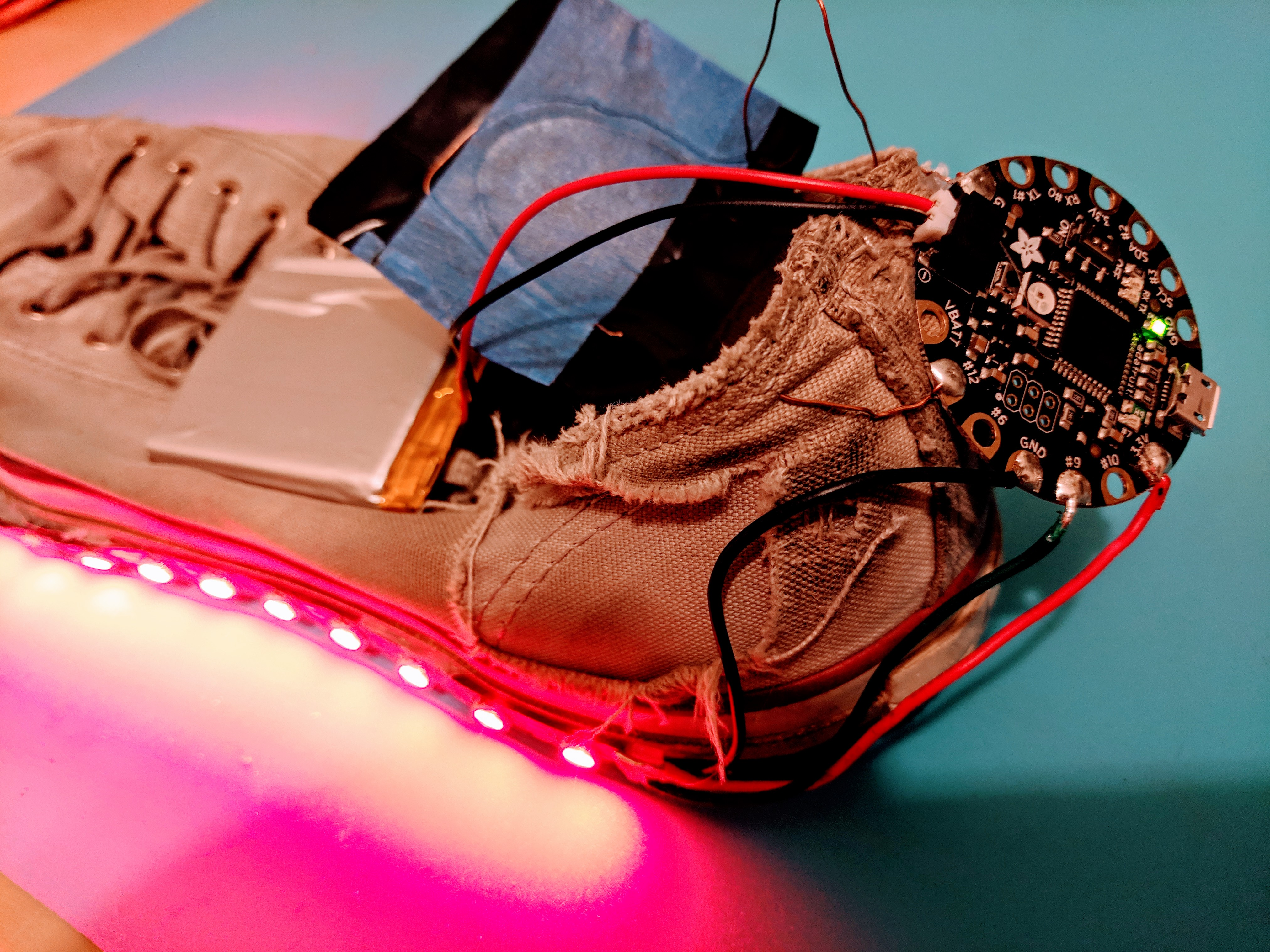The lights are LED strips controlled by an Arduino Flora using the NeoPixels library. The flora probably isn't the best microcontroller for this project, but I had them on hand, and they worked great.

The project is powered by a 3.7V 2500mA Lithium battery that lasted all day without fading color. I tested a 1200mAh current battery and it also worked fine. I bought a small microUSB charger board that worked well with the battery.

The project reads the sensorValue from a velostat with a wire on each side. As pressure is applied, the velostat changes conductivity. These values from the sensor dance around, so they get smoothed out with by only adding 10% of the new value to the old. (value = value * 0.9 + newValue * 0.1;)

The lava colors are stored in an array as just red. To make yellow sparks, green LEDs randomly light up and fade out.

materials

• a pair of shoes
• duct tape
• Adafruit FLORA - Wearable electronic platform: Arduino-compatible
• ALITOVE 16.4FT 300 Pixels WS2812B Programmable Addressable LED Strip
• velostat: ADAFRUIT INDUSTRIES 1361 Accessory Type:Conductive Sheet
• thin non-insulated copper wire
• Adafruit 328 Battery, Lithium Ion Polymer, 3.7V, 2500mAh
• Adafruit Micro Lipo w/MicroUSB Jack - USB LiIon/LiPoly charger
• Soldering required
• code

### Github - lavashoes.ino

``````#include <Adafruit_NeoPixel.h>

const int analogInPin = A11;  // A11 is called #12 on the Flora
const int NUM_LEDS = 30;
const int PIN  = 9;
const int WAIT = 10;
// adjust SENSITIVITY first, then adjust LED_OFFSET until no LEDs light up with no pressure
const int SENSITIVITY = 160;  // smaller means less lights,  bigger means more lights
const int LED_OFFSET = 56;  // adjust this to remove LEDs that stay lit up after changing sensitivity

int sensorValue = 0;
float stripLength = 0;
int color[NUM_LEDS];

void setup() {
// Serial.begin(9600);
strip.begin();
strip.show();

// set up colors array as red
for (uint16_t i = 0; i < NUM_LEDS; i++) {
color[i] = 255;
color[i] = 0;
color[i] = 0;
}
}

void loop() {
// Serial.print("sensor = " );
// Serial.println(sensorValue);

// smooth the sensorValue and scale to number of LEDs
stripLength = stripLength * 0.9 +
(SENSITIVITY * NUM_LEDS / sensorValue - LED_OFFSET) * 0.1;
//  stripLength = NUM_LEDS;//light up full strip for testing

// green spark
for (uint16_t i = 0; i < NUM_LEDS; i++) {
if (random(400) == 0 ) {
color[i] = 125;
} else if (color[i] > 1) {
color[i] -= 2;
} else {
color[i] = 0;
}
}
//light up LEDs up to stripLength
for (uint16_t i = 0; i < stripLength; i++) {
strip.setPixelColor(i, color[i], color[i], color[i]);
}
//turn off the rest of the LEDs
for (uint16_t i = stripLength; i < NUM_LEDS; i++) {
strip.setPixelColor(i, 0, 0, 0);
}
strip.show();
delay(WAIT);
}
``````

# Tron: Lightcycles

This project reproduces the tron lightcycles game. Each player controls a line of color that moves through the grid. If a player hits a location that is already lit up they die. The players will move 4x faster if the joysticks are pressed in.

After building the project I mounted it on plexiglass with screws and hotglue.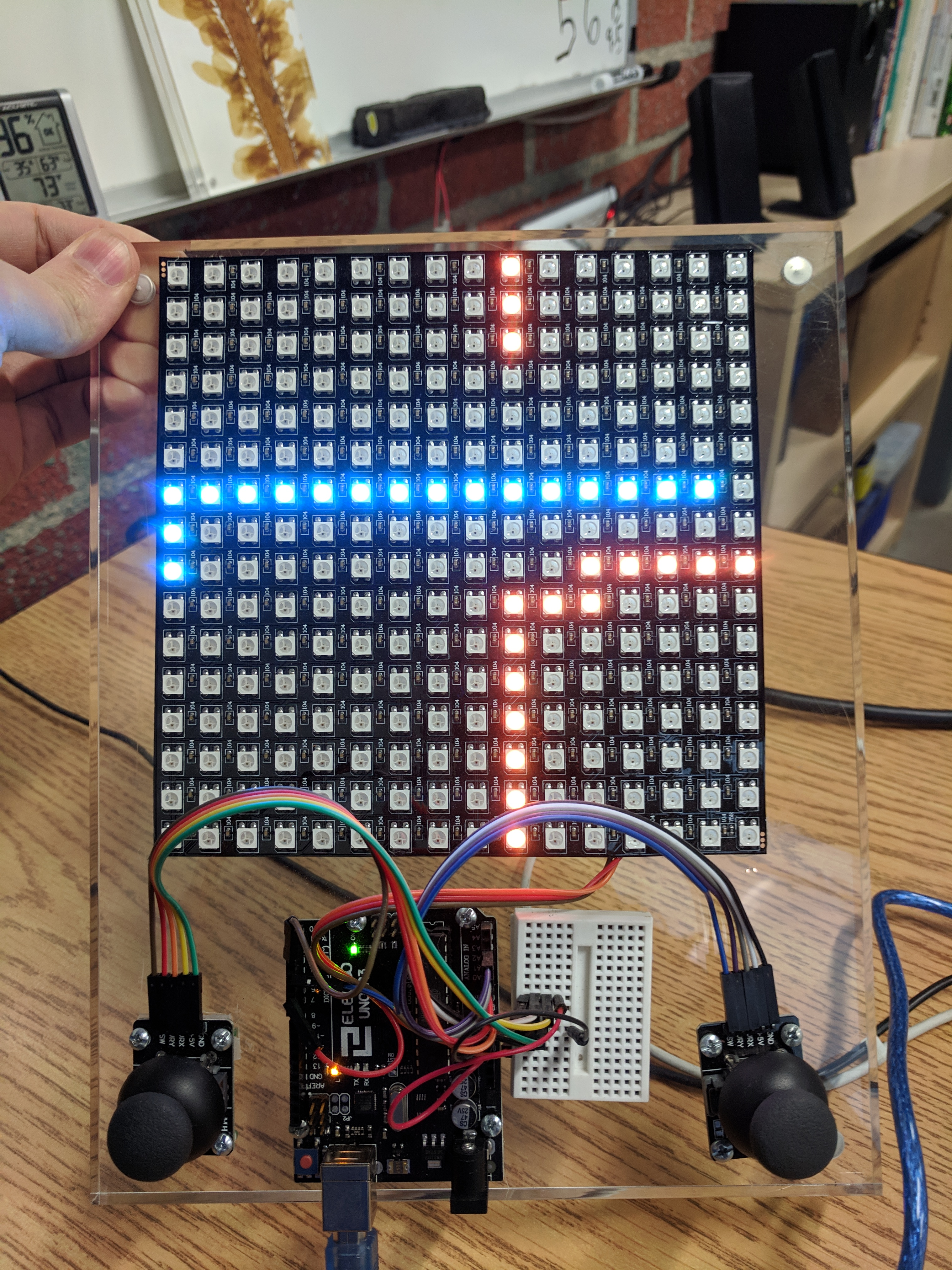The game state is stored in a 2D array of 16 x 16. The array is checked to see if a player will collide after each move.

The 16 x 16 LED matrix is organized in a zig zag pattern. I wrote a function that converts to the zigzag pattern. The NeoPixel library is used to send the final signal to light up each LED.

more pictures: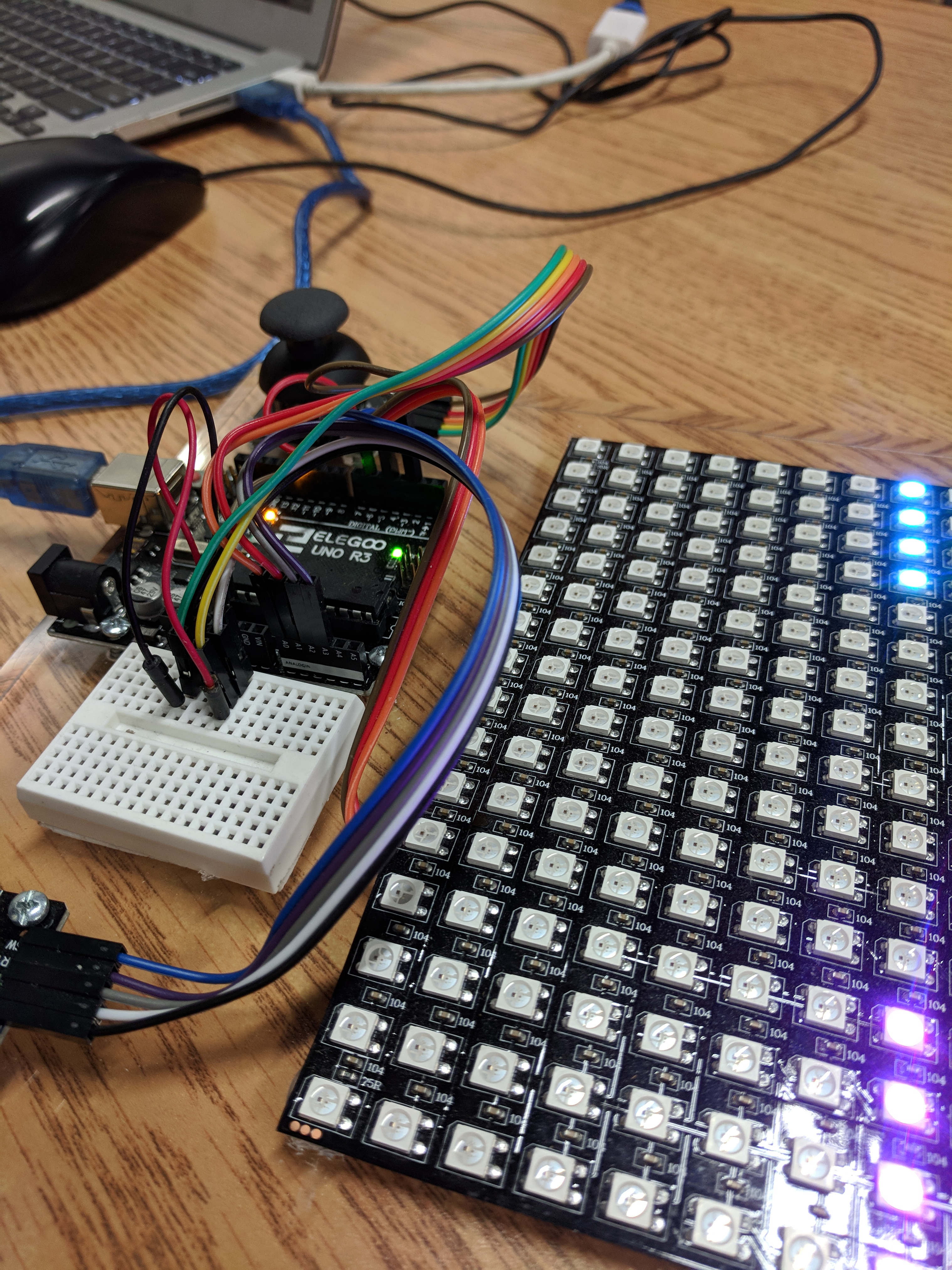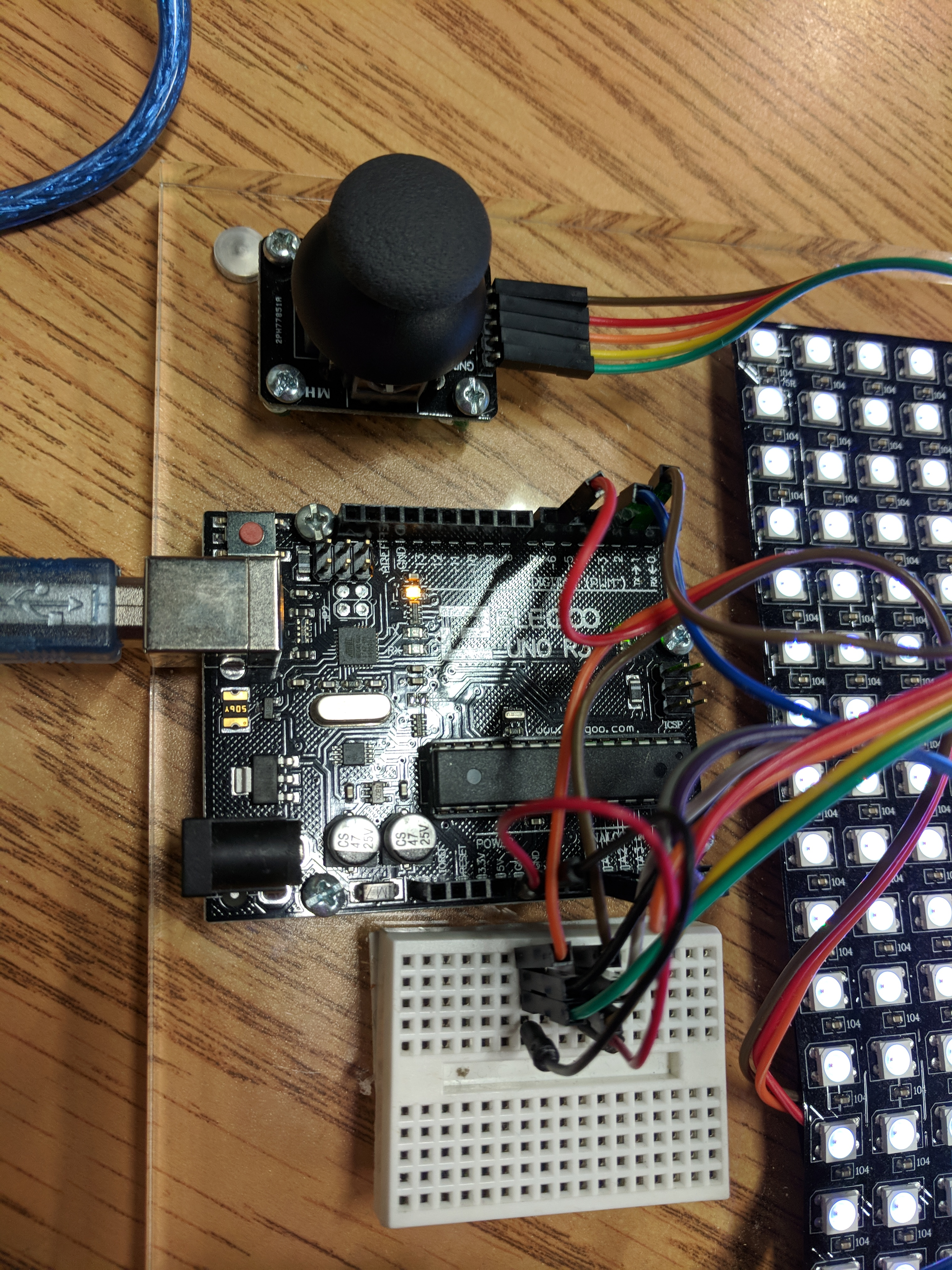materials

• Arduino Uno microcontroller
• flexible 16 x 16 NeoPixel RGB LED Matrix
• 2 Arduino Joy Sticks
• jumper wires
• code

### Github - lightcycle.ino

``````#include <Adafruit_NeoPixel.h>

const int WAIT = 250;         // how many cycles to look for player joystick input
const int LEDPIN = 6;         // pin for LEDS
const int SWITCH_PIN_1 = 2;   // pin for P1 switch
const int SWITCH_PIN_2 = 4;   // pin for P2 switch
const int NUM_LEDS = 256;     // 16x16 = 256
const int BRIGHTNESS = 30;     // 0-100 LED brightness

//player 1 is blue
//player 2 is orange

int p1 = {8, -1};  //player location
int p2 = {8, 16};   //player location
int p1Input = {0, 0};
int p2Input = {0, 0};
int p1Velocity = {0, -1};
int p2Velocity = {0, 1};
bool isP1Move = true;
bool isP2Move = true;

// locations where the players have been
bool on = {
{0, 0, 0, 0, 0, 0, 0, 0, 0, 0, 0, 0, 0, 0, 0, 0},
{0, 0, 0, 0, 0, 0, 0, 0, 0, 0, 0, 0, 0, 0, 0, 0},
{0, 0, 0, 0, 0, 0, 0, 0, 0, 0, 0, 0, 0, 0, 0, 0},
{0, 0, 0, 0, 0, 0, 0, 0, 0, 0, 0, 0, 0, 0, 0, 0},
{0, 0, 0, 0, 0, 0, 0, 0, 0, 0, 0, 0, 0, 0, 0, 0},
{0, 0, 0, 0, 0, 0, 0, 0, 0, 0, 0, 0, 0, 0, 0, 0},
{0, 0, 0, 0, 0, 0, 0, 0, 0, 0, 0, 0, 0, 0, 0, 0},
{0, 0, 0, 0, 0, 0, 0, 0, 0, 0, 0, 0, 0, 0, 0, 0},
{0, 0, 0, 0, 0, 0, 0, 0, 0, 0, 0, 0, 0, 0, 0, 0},
{0, 0, 0, 0, 0, 0, 0, 0, 0, 0, 0, 0, 0, 0, 0, 0},
{0, 0, 0, 0, 0, 0, 0, 0, 0, 0, 0, 0, 0, 0, 0, 0},
{0, 0, 0, 0, 0, 0, 0, 0, 0, 0, 0, 0, 0, 0, 0, 0},
{0, 0, 0, 0, 0, 0, 0, 0, 0, 0, 0, 0, 0, 0, 0, 0},
{0, 0, 0, 0, 0, 0, 0, 0, 0, 0, 0, 0, 0, 0, 0, 0},
{0, 0, 0, 0, 0, 0, 0, 0, 0, 0, 0, 0, 0, 0, 0, 0},
{0, 0, 0, 0, 0, 0, 0, 0, 0, 0, 0, 0, 0, 0, 0, 0},
};

// Parameter 1 = number of pixels in strip
// Parameter 2 = pin number (most are valid)
// Parameter 3 = pixel type flags, add together as needed:
//   NEO_KHZ800  800 KHz bitstream (most NeoPixel products w/WS2812 LEDs)
//   NEO_KHZ400  400 KHz (classic 'v1' (not v2) FLORA pixels, WS2811 drivers)
//   NEO_GRB     Pixels are wired for GRB bitstream (most NeoPixel products)
//   NEO_RGB     Pixels are wired for RGB bitstream (v1 FLORA pixels, not v2)

void setup() {
Serial.begin(9600);
//activate pull-up resistor on the push-button pin
pinMode(SWITCH_PIN_1, INPUT_PULLUP);
pinMode(SWITCH_PIN_2, INPUT_PULLUP);
strip.setBrightness(BRIGHTNESS);
strip.begin();
for (int i = 0; i < NUM_LEDS; i += 4) {
for (int j = 0; j < 4; j++) {
strip.setPixelColor(i + j, 255,50, 0);
strip.setPixelColor(NUM_LEDS - i - j, 0, 150, 200);
}
strip.show();
}
// clear all pixels
for (int i = 0; i < NUM_LEDS; i++) {
strip.setPixelColor(i, 0, 0, 0);
}
strip.show();
}

void loop() {
isP1Move = true;
isP2Move = true;
for (int i = 0; i < 4; i++) {
//player moves extra if button is down
isP1Move = true;
Serial.print(1);
Serial.println();
}
isP2Move = true;
Serial.print(2);
Serial.println();
}
if (isP1Move) P1Move();
if (isP2Move) P2Move();
playerJoystickInput(); //extra check for player input to smooth control sensing
playerCollisions();
updateGameState();
drawPlayers();

//check for player input
for (int i = 0; i < WAIT; i++) {
playerJoystickInput(); //consider placing playerJoystickInput() in more places in the loop to get more twitch response
}
}
}

void updateGameState() {
on[p1][p1] = true;
on[p2][p2] = true;
}

void playerJoystickInput() {
int p1x = map(analogRead(0), 0, 1023, -3, 4);
int p1y = map(analogRead(1), 0, 1023, -3, 4);
int p2x = map(analogRead(2), 0, 1023, -3, 4);
int p2y = map(analogRead(3), 0, 1023, -3, 4);

// log the input if it is not zero
if (!(p1x == 0 && p1y == 0)) {
p1Input = p1x;
p1Input = p1y;
}
if (!(p2x == 0 && p2y == 0)) {
p2Input = p2x;
p2Input = p2y;
}
}

void P1Move() {
// change player velocity from inputs
// don't let player go backwards and die
if (p1Input > 0 && p1Velocity != -1) {
p1Velocity = 1;
p1Velocity = 0;
} else if (p1Input < 0 && p1Velocity != 1) {
p1Velocity = -1;
p1Velocity = 0;
} else if (p1Input > 0 && p1Velocity != -1) {
p1Velocity = 1;
p1Velocity = 0;
} else if (p1Input < 0 && p1Velocity != 1) {
p1Velocity = -1;
p1Velocity = 0;
}

//move player 1
p1 += p1Velocity;
p1 += p1Velocity;

//edge around the world
if (p1 < 0) {
p1 = 15;
} else if (p1 > 15) {
p1 = 0;
}
if (p1 < 0) {
p1 = 15;
} else if (p1 > 15) {
p1 = 0;
}
}

void P2Move() {
// change player velocity from inputs
// don't let player go backwards and die
if (p2Input > 0 && p2Velocity != -1) {
p2Velocity = 1;
p2Velocity = 0;
} else if (p2Input < 0  && p2Velocity != 1) {
p2Velocity = -1;
p2Velocity = 0;
} else if (p2Input > 0  && p2Velocity != -1) {
p2Velocity = 1;
p2Velocity = 0;
} else if (p2Input < 0  && p2Velocity != 1) {
p2Velocity = -1;
p2Velocity = 0;
}

//move player 2
p2 += p2Velocity;
p2 += p2Velocity;

//edge around the world
if (p2 < 0) {
p2 = 15;
} else if (p2 > 15) {
p2 = 0;
}
if (p2 < 0) {
p2 = 15;
} else if (p2 > 15) {
p2 = 0;
}
}

void playerCollisions() {
//check for player + player tip collision
if (p1 == p2 && p1 == p2) {
tie();
}
//check for player1 + wall collision
if (isP1Move && on[p1][p1]) {
p2Wins();
}
//check for player2 + wall collision
if (isP2Move && on[p2][p2]) {
p1Wins();
}
//reset player moved to false
isP1Move = false;
isP2Move = false;
}

void p1Wins() {
if (isP1Move && on[p1][p1]) {
tie();
}
//  Serial.println("p1/blue wins!");
//  GameStateDump();
strip.setBrightness(20);
FillEmpty(0, 15, 20);
ZigZagPixel(p2, p2, 255, 255, 255);
strip.show();

endGame();
}

void p2Wins() {
if (isP2Move && on[p2][p2]) {
tie();
}
//  Serial.println("p2/orange, wins!");
//  GameStateDump();
strip.setBrightness(20);
FillEmpty(25, 15, 0);
ZigZagPixel(p1, p1, 255, 255, 255);
strip.show();
endGame();
}

void tie() {
//  Serial.println("tie game");
//  GameStateDump();
strip.setBrightness(20);
FillEmpty(15, 15, 15);
ZigZagPixel(p1, p1, 255, 255,255);
strip.show();
endGame();
}

void drawPlayers() {
//draw players
ZigZagPixel(p1, p1, 0, 150, 200);
ZigZagPixel(p2, p2, 255,50, 0);
strip.show();
}

void ZigZagPixel(int x, int y, int r, int g, int b) {
if (y % 2) {
strip.setPixelColor(y * 16 + x, r, g,  b);
} else {
strip.setPixelColor(y * 16 + 15 - x, r, g,  b);
}
}

void getZigZagPixel(int x, int y) {
if (y % 2) {
Serial.println(strip.getPixelColor(y * 16 + x) );
} else {
Serial.println(strip.getPixelColor(y * 16 + 15 - x));
}
}

void GameStateDump() {
for (int i = 0; i < 16; i++) {
for (int j = 0; j < 16; j++)
{
Serial.print(on[j][i]);
Serial.print(",");
}
Serial.println();
}
Serial.print("p1 = ");
Serial.print(p1);
Serial.print(", ");
Serial.print(p1);
Serial.println();
Serial.print("p2 = ");
Serial.print(p2);
Serial.print(", ");
Serial.print(p2);
Serial.println();
Serial.print("p1 Velocity = ");
Serial.print(p1Velocity);
Serial.print(", ");
Serial.print(p1Velocity);
Serial.println();
Serial.print("p2 Velocity = ");
Serial.print(p2Velocity);
Serial.print(",");
Serial.print(p2Velocity);
Serial.println();
Serial.println();
}

void FillEmpty(int r, int g, int b) {
for (int i = 0; i < 16; i++) {
for (int j = 0; j < 16; j++)
{
if (!on[i][j]) {
ZigZagPixel(i, j, r, g, b);
}
}
}
}

void(* resetFunc) (void) = 0; //declare reset function @ address 0
void endGame() {
delay(5000);
resetFunc();  //call reset
}
``````